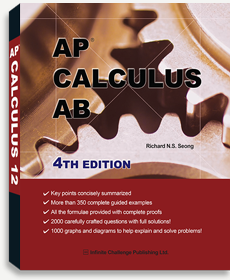Preview
ISBN: 9780991698776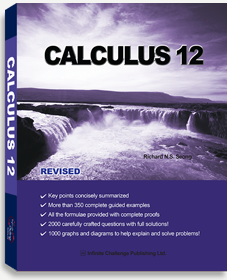Preview
ISBN: 9780991698738Preview
ISBN: 9780991698769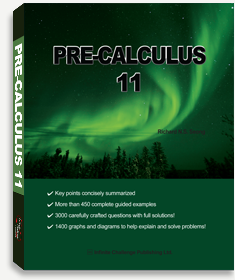Preview
ISBN: 9780991698745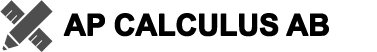• 505 Pages
• Key points concisely summarized
• More than 350 complete guided examples
• All the formulae provided with complete proofs
• 2000 carefully crafted questions with full solutions!
• 1000 graphs and diagrams to help explain and solve problems!
• About This Book
• Calculus is a branch of mathematics that is used in various fields of study such as mathematics, engineering, chemistry, physics, biology, economics, statistics, finance, etc. A solid understanding of Calculus is fundamental to both secondary school and college students.
• This book aims to implement a deeper understanding and a stronger foundation of Calculus by providing over 350 guided examples, 1600 exercise questions and 1000 detailed graphs and diagrams. Students are led step-by-step through each question, with full solutions provided at the back of the book.
• This book covers AP calculus curriculum (AB course). Thus, this book is suitable both for students approaching calculus for the first time, and those who are taking AP calculus course at a secondary school. I hope that this workbook will help students build a strong foundation of Calculus.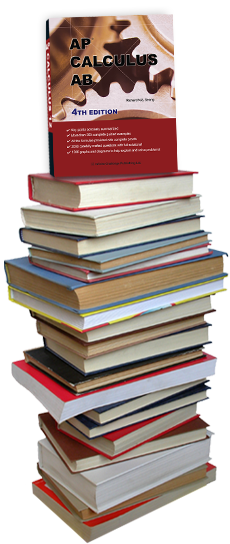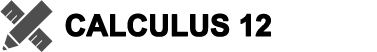• 500 Pages
• Key points concisely summarized
• More than 350 complete guided examples
• All the formulae provided with complete proofs
• 2000 carefully crafted questions with full solutions!
• 1000 graphs and diagrams to help explain and solve problems!
• About This Book
• Calculus is a branch of mathematics that is used in various fields of study such as mathematics, engineering, chemistry, physics, biology, economics, statistics, finance, etc. A solid understanding of Calculus is fundamental to both secondary school and college students.
• This book aims to implement a deeper understanding and a stronger foundation of Calculus by providing over 350 guided examples, 1600 exercise questions and 1000 detailed graphs and diagrams. Students are led step-by-step through each question, with full solutions provided at the back of the book.
• This book is suitable for both secondary school and college students approaching Calculus for the first time. I hope that this workbook will help students build a strong foundation of Calculus.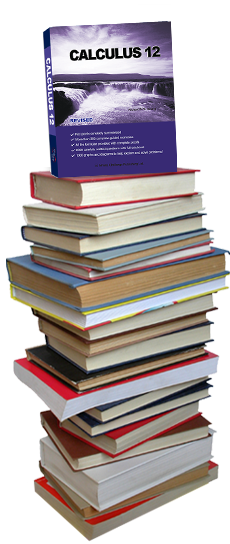no images were found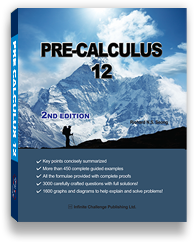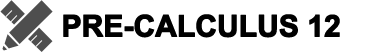• 520 Pages
• Key points concisely summarized
• More than 450 complete guided examples
• All the formulae provided with complete proofs
• 3000 carefully crafted questions with full solutions!
• 1600 graphs and diagrams to help explain and solve problems!
• About This Book
• Pre-calculus is a math course that equips the students with the mathematical concepts, techniques and insights required to understand Calculus – mathematics used in various fields of study such as mathematics, engineering, chemistry, physics, biology, economics, statistics, finance, etc.
• This book aims to implement a deeper understanding and a stronger foundation of Pre-calculus by providing over 450 guided examples, 2700 exercise questions and 1600 detailed graphs and diagrams. Students are led step-by-step through each question, with full solutions provided at the back of the book.
• I hope that this workbook will help students build a strong foundation of Pre-calculus.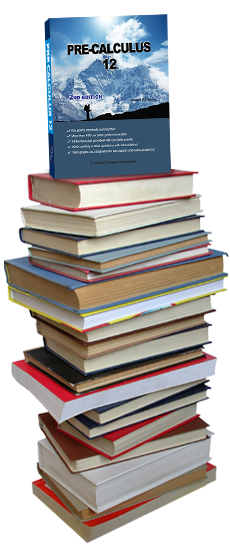• 522 Pages
• Key points concisely summarized
• More than 450 complete guided examples
• 3000 carefully crafted questions with full solutions!
• 1400 graphs and diagrams to help explain and solve problems!
• About This Book
• Pre-calculus is a math course that equips the students with the mathematical concepts, techniques and insights required to understand Calculus – mathematics used in various fields of study such as mathematics, engineering, chemistry, physics, biology, economics, statistics, finance, etc.
• This book aims to implement a deeper understanding and a stronger foundation of Pre-calculus by providing over 450 guided examples, 2700 exercise questions and 1400 detailed graphs and diagrams. Students are led step-by-step through each question, in the full solutions provided at the back of the book.
• I hope that this workbook will help students build a strong foundation of Pre-calculus.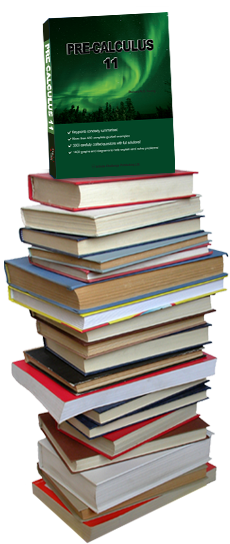“AP® is a trademark registered by the College Board, which was not involved in the production of, and does not endorse, this site.”